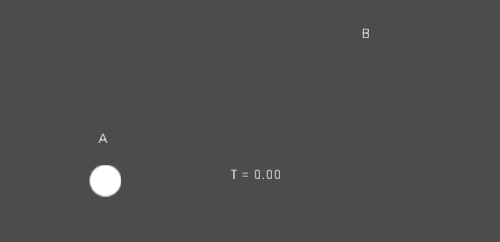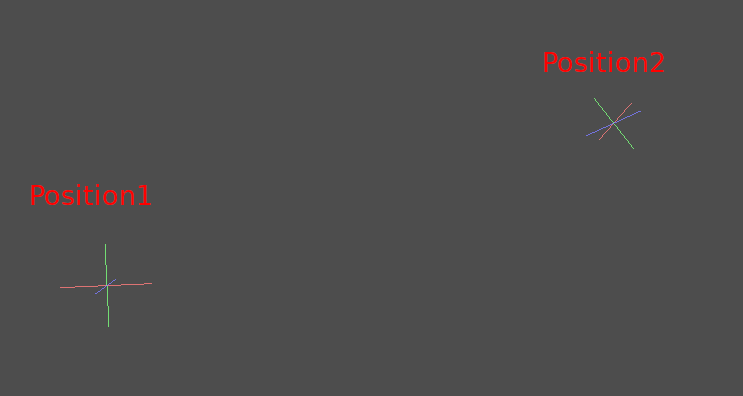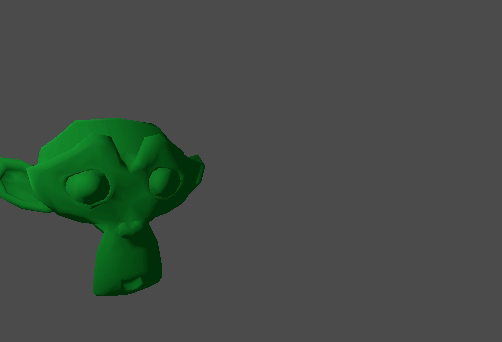# Interpolation¶

Interpolation is a very basic operation in graphics programming. It’s good to become familiar with it in order to expand your horizons as a graphics developer.

The basic idea is that you want to transition from A to B. A value `t`, represents the states in-between.

As an example if `t` is 0, then the state is A. If `t` is 1, then the state is B. Anything in-between is an interpolation.

Between two real (floating point) numbers, a simple interpolation is usually described as:

```interpolation = A * (1 - t) + B * t
```

And often simplified to:

```interpolation = A + (B - A) * t
```

which is exactly the same.

The name of this type of interpolation, which transforms a value into another at constant speed is « linear ». So, when you hear about Linear Interpolation, you know they are referring to this simple formula.

There are other types of interpolations, which will not be covered here. A recommended read afterwards is the Bezier page.

## Interpolation vectorielle¶

Vector types (Vector2 and Vector3) can also be interpolated, they come with handy functions to do it Vector2.linear_interpolate() and Vector3.linear_interpolate().

For cubic interpolation, there are also Vector2.cubic_interpolate() and Vector3.cubic_interpolate(), which do a Bezier style interpolation.

Here is simple pseudo-code for going from point A to B using interpolation:

```func _physics_process(delta):
t += delta * 0.4

\$Sprite.position = \$A.position.linear_interpolate(\$B.position, t)
```

Il produira le mouvement suivant :## Interpolation par transformation¶

It is also possible to interpolate whole transforms (make sure they have either uniform scale or, at least, the same non-uniform scale). For this, the function Transform.interpolate_with() can be used.

Here is an example of transforming a monkey from Position1 to Position2:En utilisant le pseudo-code suivant :

```var t = 0.0

func _physics_process(delta):
t += delta

\$Monkey.transform = \$Position1.transform.interpolate_with(\$Position2.transform, t)
```

And again, it will produce the following motion:## Mouvement lisser¶

Interpolation can be used to smooth movement, rotation, etc. Here is an example of a circle following the mouse using smoothed motion:

```const FOLLOW_SPEED = 4.0

func _physics_process(delta):
var mouse_pos = get_local_mouse_position()

\$Sprite.position = \$Sprite.position.linear_interpolate(mouse_pos, delta * FOLLOW_SPEED)
```

Voici à quoi cela ressemble :This useful for smoothing camera movement, allies following you (ensuring they stay within a certain range), and many other common game patterns.